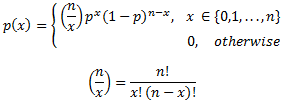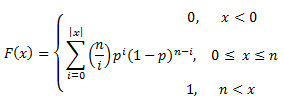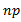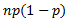AnyLogic

# binomial

 Probability mass functionDistributionMeanVarianceThe Binomial distribution is a discrete distribution bounded by [0,n]. Typically, it is used where a single trial is repeated over and over, such as the tossing of a coin. The parameter, p, is the probability of the event, either heads or tails, either occurring or not occurring. Each single trial is assumed to be independent of all others. For large n, the Binomial distribution may be approximated by the Normal distribution, for example when np > 9 and p < 0.5 or when np(1-p) > 9.

As shown in the examples above, low values of p give high probabilities for low values of x and visa versa, so that the peak in the distribution may approach either bound. The Binomial distribution has had extensive use in games, but is also useful in genetics, sampling of defective parts in a stable process, and other event sampling tests where the probability of the event is known to be constant or nearly so.

### binomial(double p, int n)

Description
Generates a sample of the Binomial distribution.
Parameters
Name Type of value Description
p double The probability of the event occurrence.
n int The number of trials.
Result
Type Description
int The generated sample.

### binomial(double p)

Description
Generates a sample of the Binomial distribution with n set to 1. Is equivalent to binomial(dd, 1).
Parameters
Name Type of value Description
p double The probability of the event occurrence.
Result
Type Description
int The generated sample.

### binomial(double p, int n, java.util.Random r)

Description
Generates a sample of the Binomial distribution using the specified random number generator.
Parameters
Name Type of value Description
p double The probability of the event occurrence.
n int The number of trials.
r java.util.Random The random number generator.
Result
Type Description
int The generated sample.

This document includes content from the “Stat::Fit User’s Manual”. Copyright 2016 Geer Mountain Software Corp.